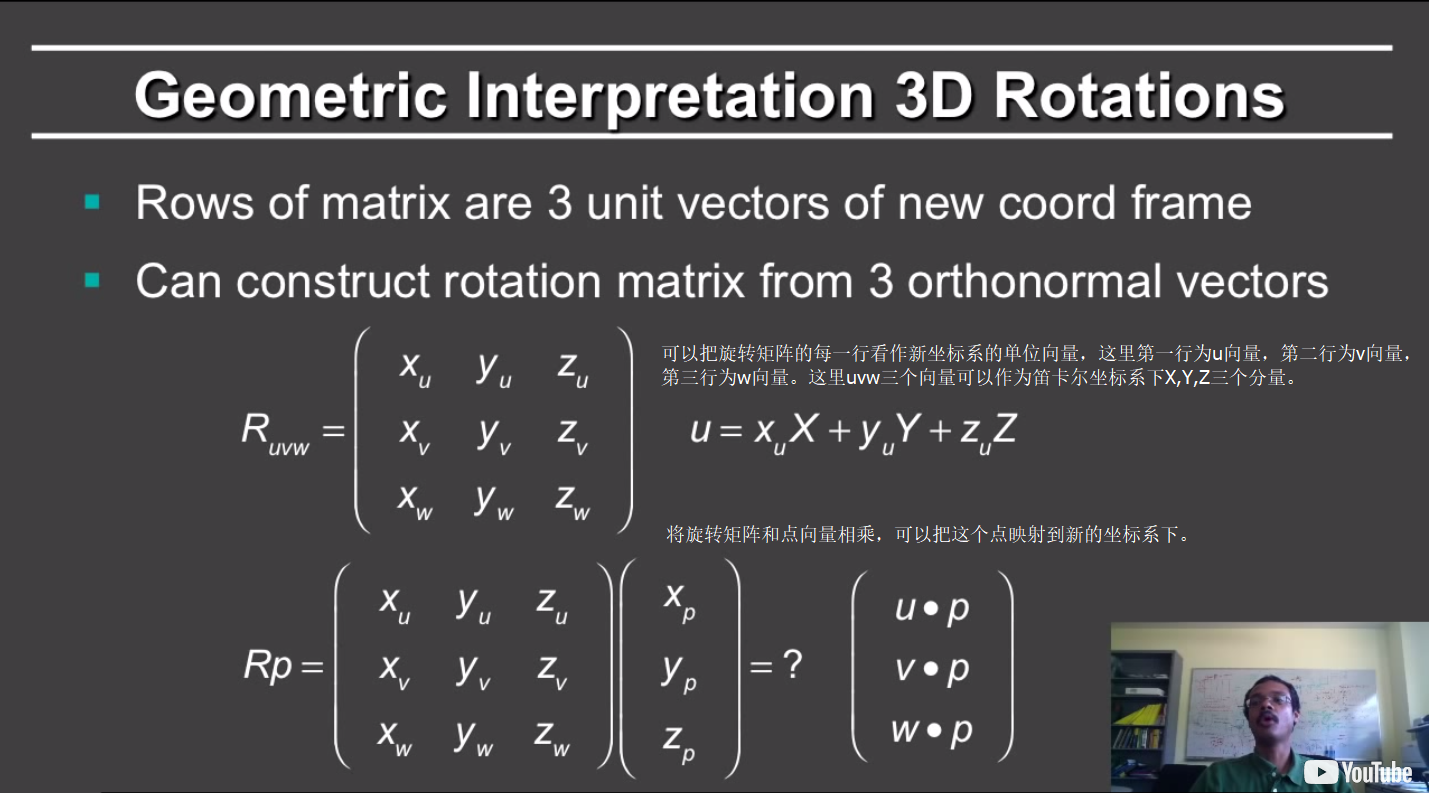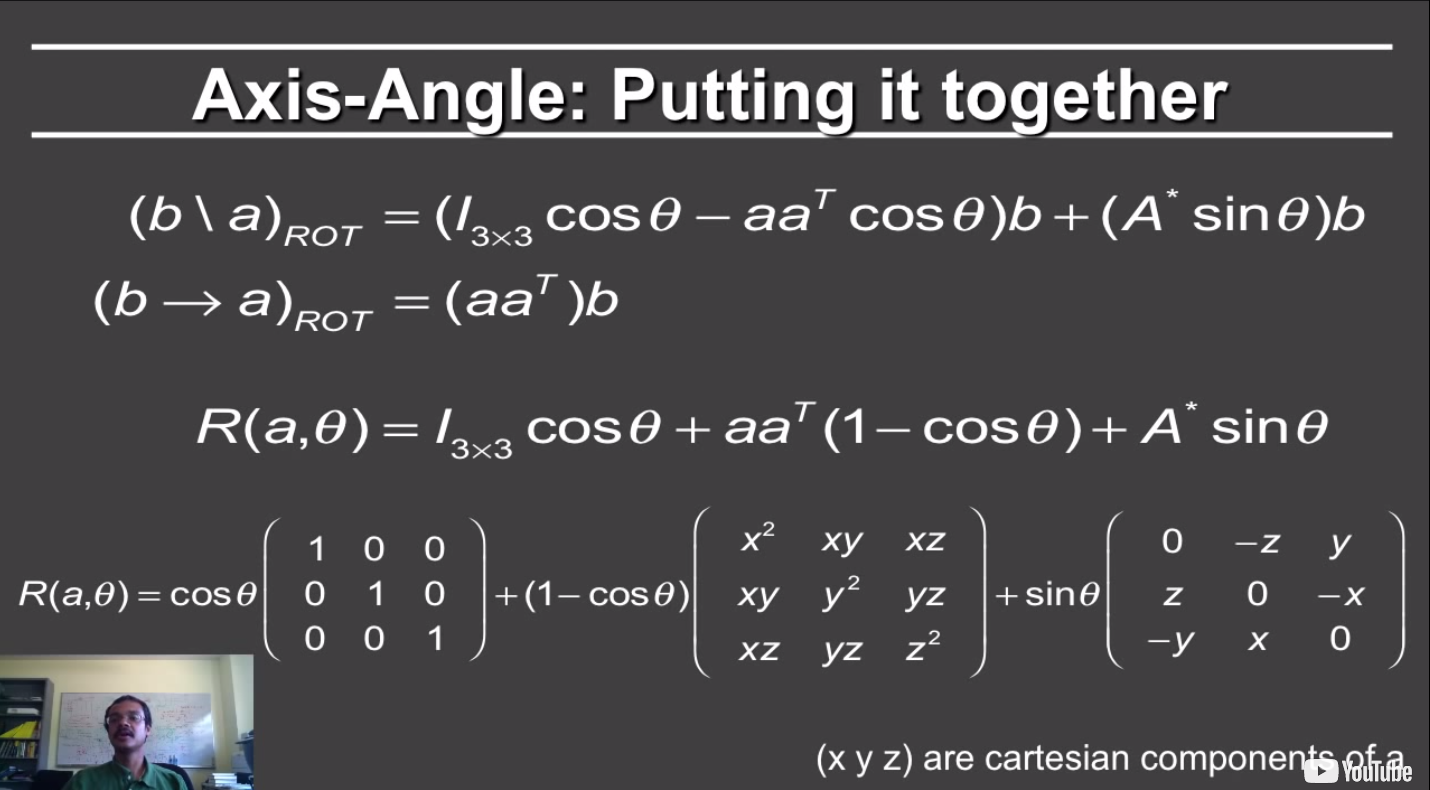2020年7月26日更新：

## 基础运算

### 点积（Dot Product）

• 求两个向量之间的夹角（光源和表面之间夹角的余弦值对于投影来说非常重要）
• 找到一个向量在另一向量上的投影也非常重要（比如我们想知道一个点在新的坐标系下的坐标）。
• 点积在笛卡尔坐标系下很有用

$\vec{a} \cdot \vec{b}=\|a\|\|b\| \cos \theta$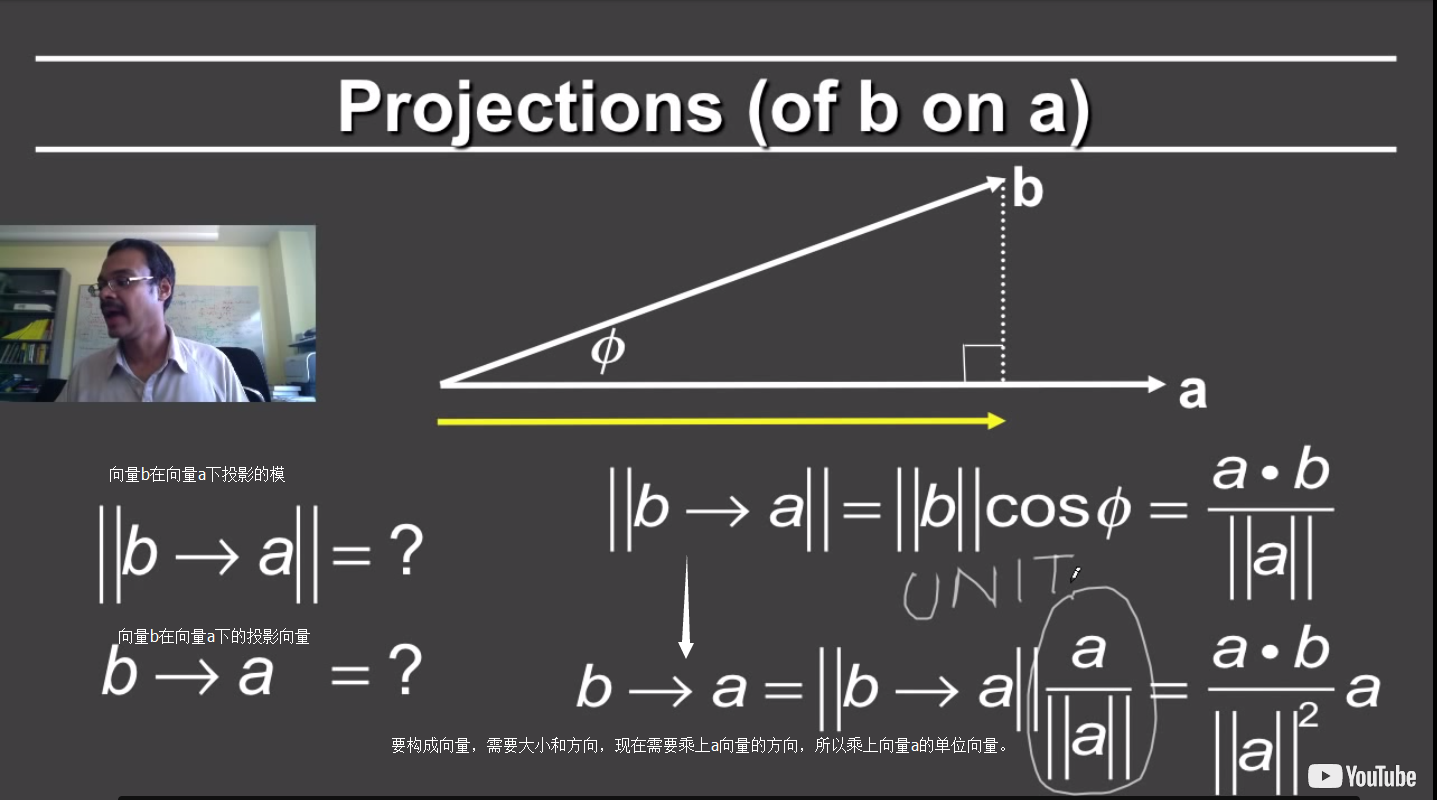### 叉积（Cross Product）

$\begin{array}{l}{a \times b=-b \times a} \\ {\|a \times b\|=\|a\|\|b\| \sin \theta}\end{array}$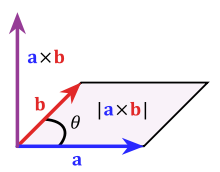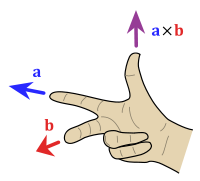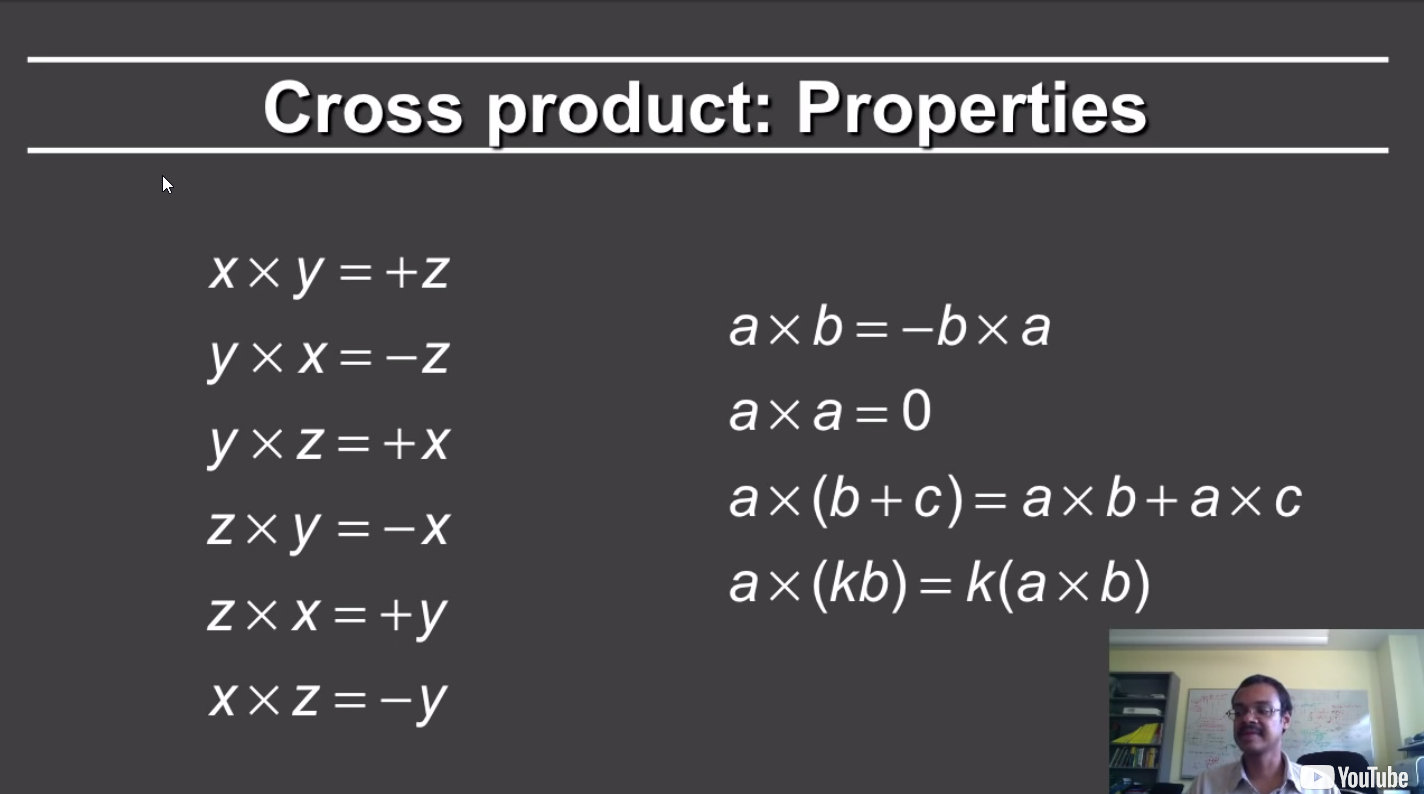### 正交坐标系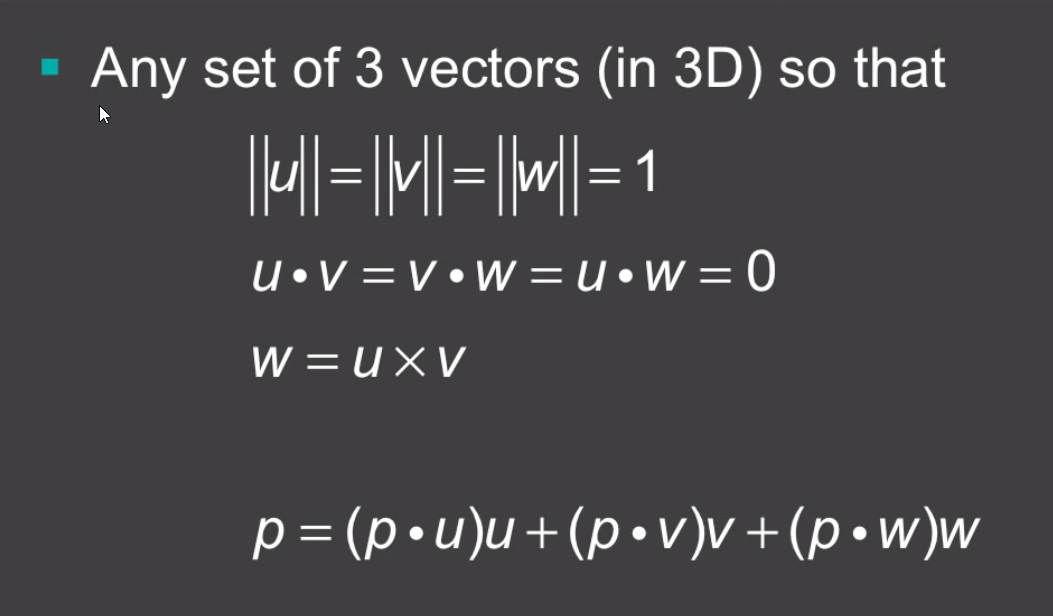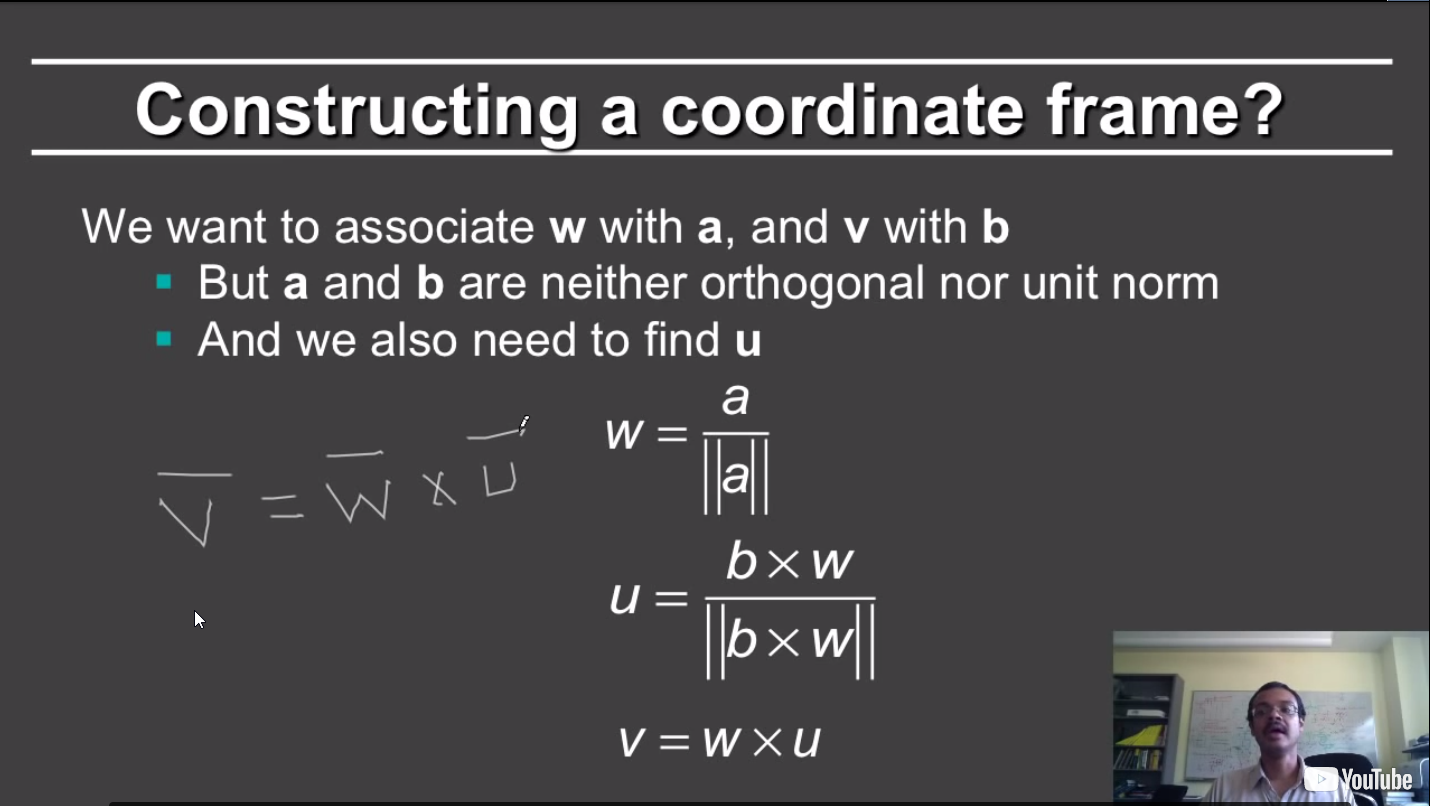### 矩阵

#### 矩阵相乘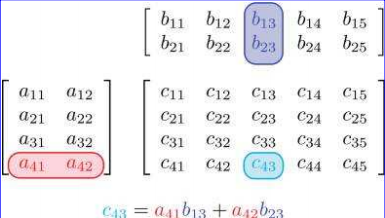#### 矩阵转置

$\left[\begin{array}{ccc}{1} & {2} & {3} \\ {4} & {5} & {6} \\ {7} & {8} & {9} \\ {10} & {11} & {12}\end{array}\right]^{T}=\left[\begin{array}{cccc}{1} & {4} & {7} & {10} \\ {2} & {5} & {8} & {11} \\ {3} & {6} & {9} & {12}\end{array}\right]$

$\left[\begin{array}{lll}{a} & {b} & {c} \\ {d} & {e} & {f} \\ {g} & {h} & {i}\end{array}\right]^{T}=\left[\begin{array}{lll}{a} & {d} & {g} \\ {b} & {e} & {h} \\ {c} & {f} & {i}\end{array}\right]$

$\left[\begin{array}{lll}{x} & {y} & {z}\end{array}\right]^{T}=\left[\begin{array}{l}{x} \\ {y} \\ {z}\end{array}\right] \quad\left[\begin{array}{l}{x} \\ {y} \\ {z}\end{array}\right]^{T}=\left[\begin{array}{lll}{x} & {y} & {z}\end{array}\right]$

$(\mathbf{A B})^{T}=\mathbf{B}^{\mathrm{T}} \mathbf{A}^{\mathrm{T}}$

#### 矩阵的逆

$\left(\mathbf{M}^{\mathrm{T}}\right)^{-1}=\left(\mathbf{M}^{-1}\right)^{\mathrm{T}}$

$\left(\mathbf{M}_{1} \mathbf{M}_{2} \cdots \mathbf{M}_{n-1} \mathbf{M}_{n}\right)^{-1}=\mathbf{M}_{n}^{-1} \mathbf{M}_{n-1}^{-1} \cdots \mathbf{M}_{2}^{-1} \mathbf{M}_{1}^{-1}$

$\left|\mathbf{M}^{-1}\right|=1 /|\mathbf{M}|$

$\mathbf{M}\left(\mathbf{M}^{-1}\right)=\mathbf{M}^{-1} \mathbf{M}=\mathbf{I}$

## 矩阵变换

### 缩放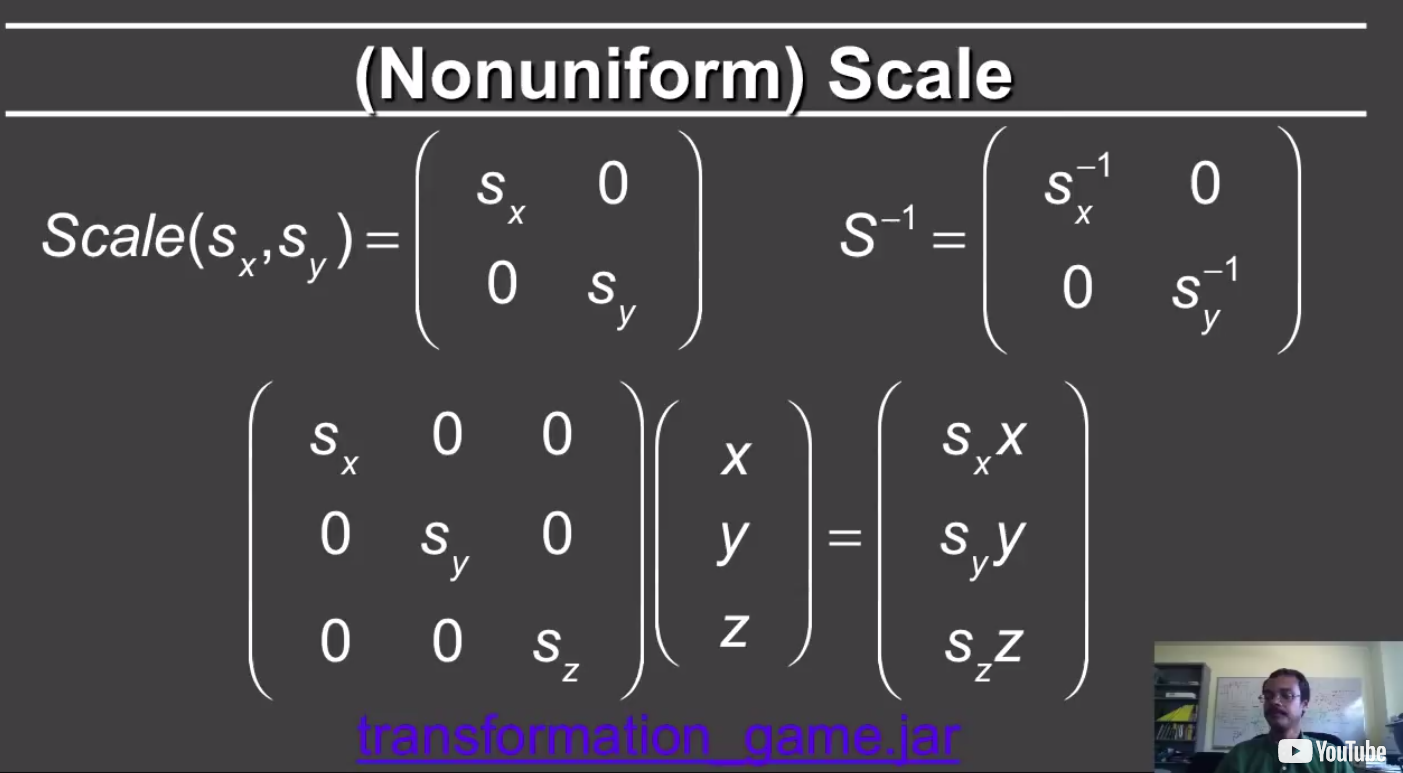### 切变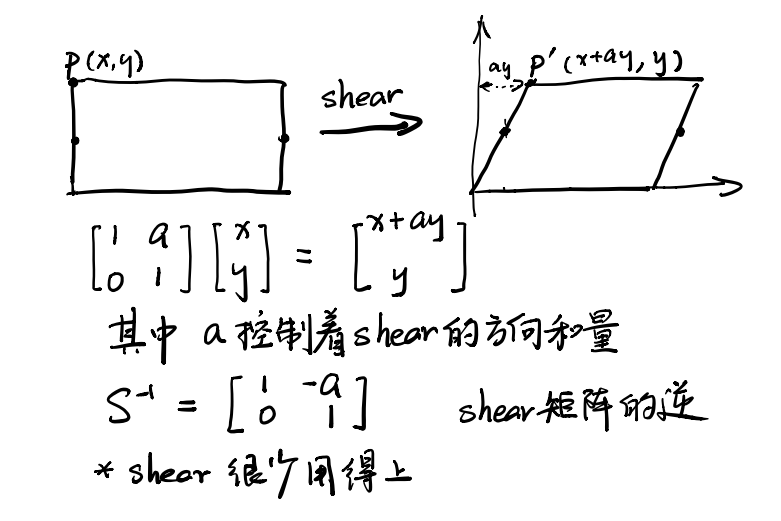### 旋转

#### 二维空间下的旋转#### 三维空间下的旋转##### 绕任意轴旋转